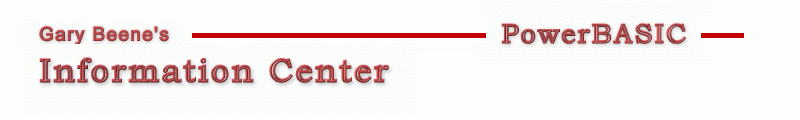## Numeric Sort

Category: Sorting

Date: 03-28-2012

'PB Array statements have built-in capability to manipulate arrays

'Primary Code:
'Syntax (Numeric Array):
'ARRAY SORT darray([index]) [FOR count] [,TAGARRAY tarray()] [,{ASCEND | DESCEND}]

'All or some elements of an array can be sorted, in ascending/descending order
'Tag-along array elements are swapped in the same order as the sorted array

Array Sort MyArray()                   'sorts entire array, ascending (default)
Array Sort MyArray(), Descend          'sorts entire array, descending

Array Sort MyArray(5)                  'sorts array from 5 to UBound(MyArray), ascending
Array Sort MyArray(5), Descend         'sorts array from 5 to UBound(MyArray), descending

Array Sort MyArray(5) For 10           'sorts elements 5-14, ascending
Array Sort MyArray(5), For 10 Descend  'sorts elements 5-14, descending

Array Sort MyArray()                   'sorts entire array, ascending
Array Sort MyArray(), Descend          'sorts entire array, descending

Array Sort MyArray(), TAGARRAY MyTagArray()           'sorts entire array, ascending (default). MyTagArray() elements swapped same as MyArray()
Array Sort MyArray(), TAGARRAY MyTagArray(), Descend  'sorts entire array, descending.  MyTagArray() elements swapped same as MyArray()

'Compilable Example:
'The first button simply sorts a numeric array. The second button sorts a numeric
'array in descending order and shows the results of a string tagarray as well.
#Compiler PBWin 9, PBWin 10
#Compile EXE
#Dim All
#Include "Win32API.inc"
Global hDlg As DWord

Function PBMain() As Long
Dialog New Pixels, 0, "Test Code",300,300,200,200, %WS_OverlappedWindow To hDlg
Control Add Button, hDlg, 100,"Push", 50,10,100,20
Control Add Button, hDlg, 200,"Push", 50,40,100,20
Dialog Show Modal hDlg Call DlgProc
End Function

CallBack Function DlgProc() As Long
Dim MyArray(9) as Long, tempA\$, tempB\$, tempC\$,i as Long, MyTagArray(9) as String
Array Assign MyArray() = 0,5,3,6,4,2,9,7,8,1
Array Assign MyTagArray() = "A","F","d","G","e","C","J","h","i","B"
For i = 0 to 9 : tempA\$ = tempA\$ + Str\$(MyArray(i)) + " " : Next i
If CB.Msg = %WM_Command AND CB.Ctl = 100 AND CB.Ctlmsg = %BN_Clicked Then
Array Sort MyArray()
For i = 0 to 9 : tempB\$ = tempB\$ + Str\$(MyArray(i)) + " " : Next i
For i = 0 to 9 : tempC\$ = tempC\$ + MyTagArray(i) + " " : Next i
MsgBox "start:" + \$crlf + tempA\$ + \$crlf + \$crlf + "result:" + \$crlf + tempB\$
End If
If CB.Msg = %WM_Command AND CB.Ctl = 200 AND CB.Ctlmsg = %BN_Clicked Then
Array Sort MyArray(), TAGARRAY MyTagArray(), Descend    'sorts entire array, descending.  MyTagArray() elements swapped same as MyArray()
For i = 0 to 9 : tempB\$ = tempB\$ + Str\$(MyArray(i)) + " " : Next i
tempC\$ = Join\$(MyTagArray(), " ")
MsgBox "start:" + \$crlf + tempA\$ + \$crlf + \$crlf + "result:" + \$crlf + tempB\$ + \$crlf + \$crlf + "tagarray:" + \$crlf + tempC\$
End If
End Function

'gbs_00235
'Date: 03-10-2012

created by gbSnippets
http://www.garybeene.com/sw/gbsnippets.htm